Matthew Kiss

•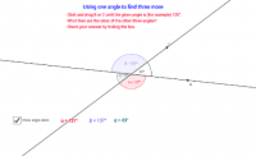vertically opposite angles _example

Activity

Matthew Kiss

•vertically opposite angles

Activity

Matthew Kiss

•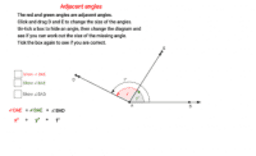Activity

Matthew Kiss

•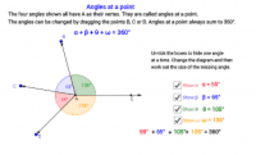angles at a point

Activity

Matthew Kiss

•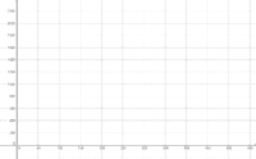linear functions_break even point

Activity

Matthew Kiss

•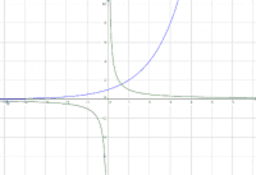non linear functions

Activity

Matthew Kiss

•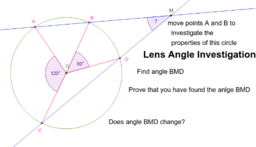Circle geometry - Lens angle investigation

Activity

Matthew Kiss

•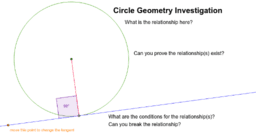Circle Geometry - Tangent to a circle

Activity

Matthew Kiss

•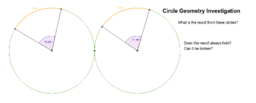circle geometry - angles and arc lengths of equal circles

Activity

Matthew Kiss

•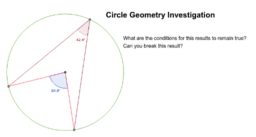Circle geometry - angle at centre and angle at circumference

Activity

Matthew Kiss

•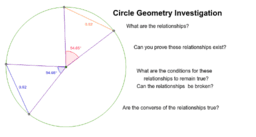Equal angles and equal chords on a circle

Activity

Matthew Kiss

•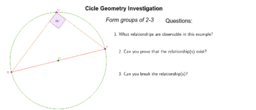Circle geometry - angle in a semi-circle

Activity

Matthew Kiss

•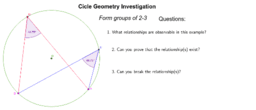Circle geometry - Angles in a segment

Activity

Matthew Kiss

•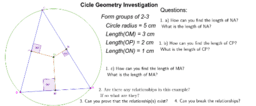Circle geometry - concyclic points

Activity

Matthew Kiss

•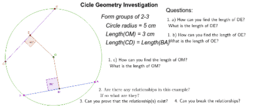Circle geometry - equal chords in a circle

Activity

Matthew Kiss

•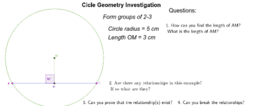circle geometry - perpendicular to the centre from a chord

Activity

Matthew Kiss

•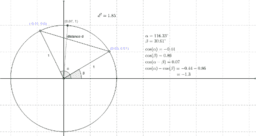Trigonometric difference relation investigation

Activity

Matthew Kiss

•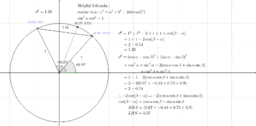Trigonometric difference relation

Activity

Matthew Kiss

•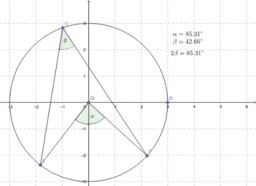Double angle at the centre of a circle

Activity

Matthew Kiss

•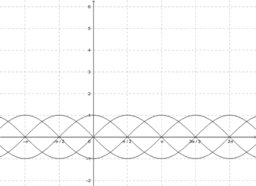sine and cosine shifted out of phase

Activity

Matthew Kiss

•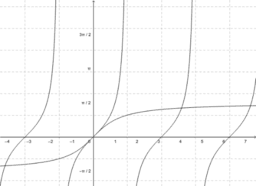inverse trig functions

Activity

Matthew Kiss

•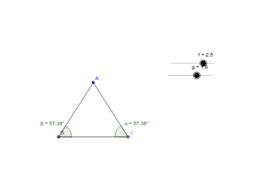isosceles triangle

Activity

Matthew Kiss

•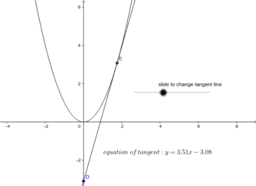tangent parabola

Activity

Matthew Kiss

•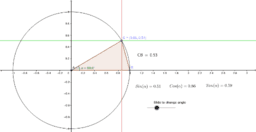Activity

Matthew Kiss